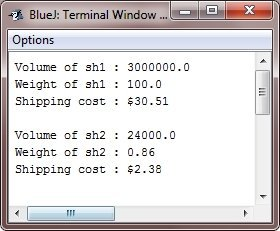# Java Multilevel Hierarchy

In Java, you can build the hierarchies that contain as many layers of inheritance as you like.

As mentioned, it is absolutely acceptable to use a subclass as a superclass of another. For example, given three class A, B, and C, the class C can be a subclass of B, which is a subclass of A. When this type of situation occurs, each subclass inherits all of the traits which found in all of its superclasses. In this case, C inherits all the aspects of B and A. To see how a multilevel hierarchy can be useful, now look at the following example program. In it, the subclass BoxWeight is used as a superclass to create a subclass named Shipment. The Shipment inherits all of the traits of the BoxWeight and Box, and adds a field named cost, which holds the cost of shipping such a parcel.

## Java Multilevel Hierarchy Example

Here is an example program, illustrates multilevel hierarchy in Java:

```/* Java Program Example - Java Create Multilevel Hierarchy
* Extend the BoxWeight to include shipping costs
*/

class Box
{
private double box_width;
private double box_height;
private double box_depth;

/* construct the clone of an object */
Box(Box obj)      // pass the object to the constructor
{
box_width = obj.box_width;
box_height = obj.box_height;
box_depth = obj.box_depth;
}

/* constructor used when all the dimensions specified */
Box(double wei, double hei, double dep)
{
box_width = wei;
box_height = hei;
box_depth = dep;
}

/* constructor used when no dimensions specified */
Box()
{
box_width = -1;          // use -1 to indicate
box_height = -1;         // an uninitialized
box_depth = -1;          // box
}

/* constructor used when cube is created */
Box(double len)
{
box_width = box_height = box_depth = len;
}

/* compute and return volume */
double volume()
{
return box_width * box_height * box_depth;
}
}

class BoxWeight extends Box
{
double weight;      // weight of box

/* construct the clone of an object */
BoxWeight(BoxWeight obj)           // pass the object to constructor
{
super(obj);
weight = obj.weight;
}

/* constructor, when all the parameters are specified */
BoxWeight(double wei, double hei, double dep, double m)
{
super(wei, hei, dep);       // call the superclass constructor
weight = m;
}

/* default constructor */
BoxWeight()
{
super();
weight = -1;
}

/* constructor used when cube is created */
BoxWeight(double len, double m)
{
super(len);
weight = m;
}
}

/* now add the shipping costs */
class Shipment extends BoxWeight
{
double cost;

/* construct clone of an object */
Shipment(Shipment obj)       // pass the object to the constructor
{
super(obj);
cost = obj.cost;
}

/* constructor when all the parameters are specified */
Shipment(double wei, double hei, double dep, double m, double cos)
{
super(wei, hei, dep, m);     // call the superclass constructor
cost = cos;
}

/* default constructor */
Shipment()
{
super();
cost = -1;
}

/* constructor used when the cube is created */
Shipment(double len, double m, double cos)
{
super(len, m);
cost = cos;
}
}

class JavaProgram
{
public static void main(String args[])
{
Shipment sh1 = new Shipment(100, 200, 150, 100, 30.51);
Shipment sh2 = new Shipment(20, 30, 40, 0.86, 2.38);

double vol;

/* get the volume of the sh1 */
vol = sh1.volume();

/* print volume, weight, and shipping cost of sh1 */
System.out.println("Volume of sh1 : " + vol);
System.out.println("Weight of sh1 : " + sh1.weight);
System.out.println("Shipping cost : \$" + sh1.cost);
System.out.println();

/* get the volume of the sh2 */
vol = sh2.volume();

/* print volume, weight, and shipping cost of sh2 */
System.out.println("Volume of sh2 : " + vol);
System.out.println("Weight of sh2 : " + sh2.weight);
System.out.println("Shipping cost : \$" + sh2.cost);
}
}```

When the above Java program is compile and executed, it will produce the following output:Because of inheritance, Shipment can make use of previously defined classes of the Box and BoxWeight, adding only extra information it needs for its own, specific application. This is the part of the value of inheritance, it allows the reuse of code.

Tools
Calculator